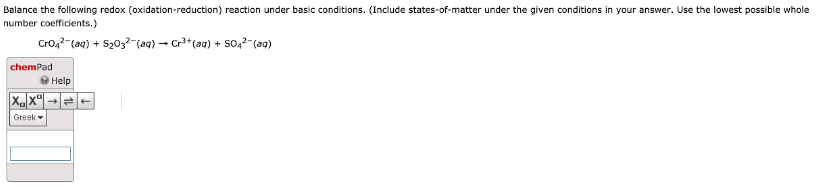# Balance the following redox (oxidation-reduction) reaction under basic conditions. (Include states-of-matter under the given conditions in your answer. Use the lowest possible whole number coefficients.) CrO4^2-(aq) + S2O3^2-(aq) -> Cr3+(aq) + SO4^2-(aq)